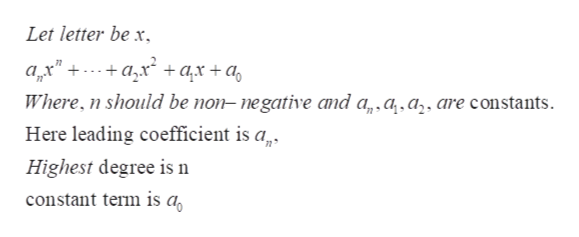# Indicate whether the expression defines a polynomial function.R(x) = 1/x  +6If it is a polynomial function, identify the following. (If it is not a polynomial function, enter DNE for all three answers.)(a) Identify the leading coefficient.  (b) Identify the constant term.  (c) State the degree.

Question

Indicate whether the expression defines a polynomial function.

R(x) = 1/x  +6
If it is a polynomial function, identify the following. (If it is not a polynomial function, enter DNE for all three answers.)

(b) Identify the constant term.

(c) State the degree.

check_circle

Step 1

Refer to the question, we have to identify whether the given function R(x) = 1/x  +6

is polynomial or not.

Step 2

Now according to the definition of polynomial it is an algebraic expression that is sum...help_outlineImage TranscriptioncloseLet letter be x ax" a+ ax + a Where, n should be non- negative and a,a.a,, are constants Here leading coefficient is a Highest degree is n constant term is a fullscreen

### Want to see the full answer?

See Solution

#### Want to see this answer and more?

Solutions are written by subject experts who are available 24/7. Questions are typically answered within 1 hour.*

See Solution
*Response times may vary by subject and question.
Tagged in

### Algebra